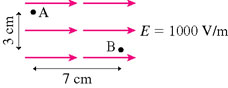# Potential Difference (physics)?

edited

Potential Difference (physics)?Which point, A or B, has a larger electric potential?

What is the potential difference between A and B?

Update:

by
selected by

The potential difference between two points, A & B, in an E-field is the negative of the work per unit charge to move a charge from A to B.

V(B) - V(A) = - (1/q)Work Done by E (A to B)

You can take any path from A to B . The easiest path is from A straight down 3 cm (the work is zero on this path because the displacement is perpendicular to force qE). Then straight across 7cm to B. (the work is qEX = qE(.07) for this part since the force is parallel to the displacement).

V(B) - V(A) = - (1/q)(0) - (1/q)qE(.07)

V(B) - V(A) = - (.07)E

Since the difference is negative it means V(A) > V(B)

Lorem ipsum dolor sit amet, consectetur adipiscing elit, sed do eiusmod tempor incididunt ut labore et dolore magna aliqua. Ut enim ad minim veniam, quis nostrud exercitation ullamco laboris nisi ut aliquip ex ea commodo consequat. Duis aute irure dolor in reprehenderit in voluptate velit esse cillum dolore eu fugiat.

+1 vote
+1 vote
+1 vote